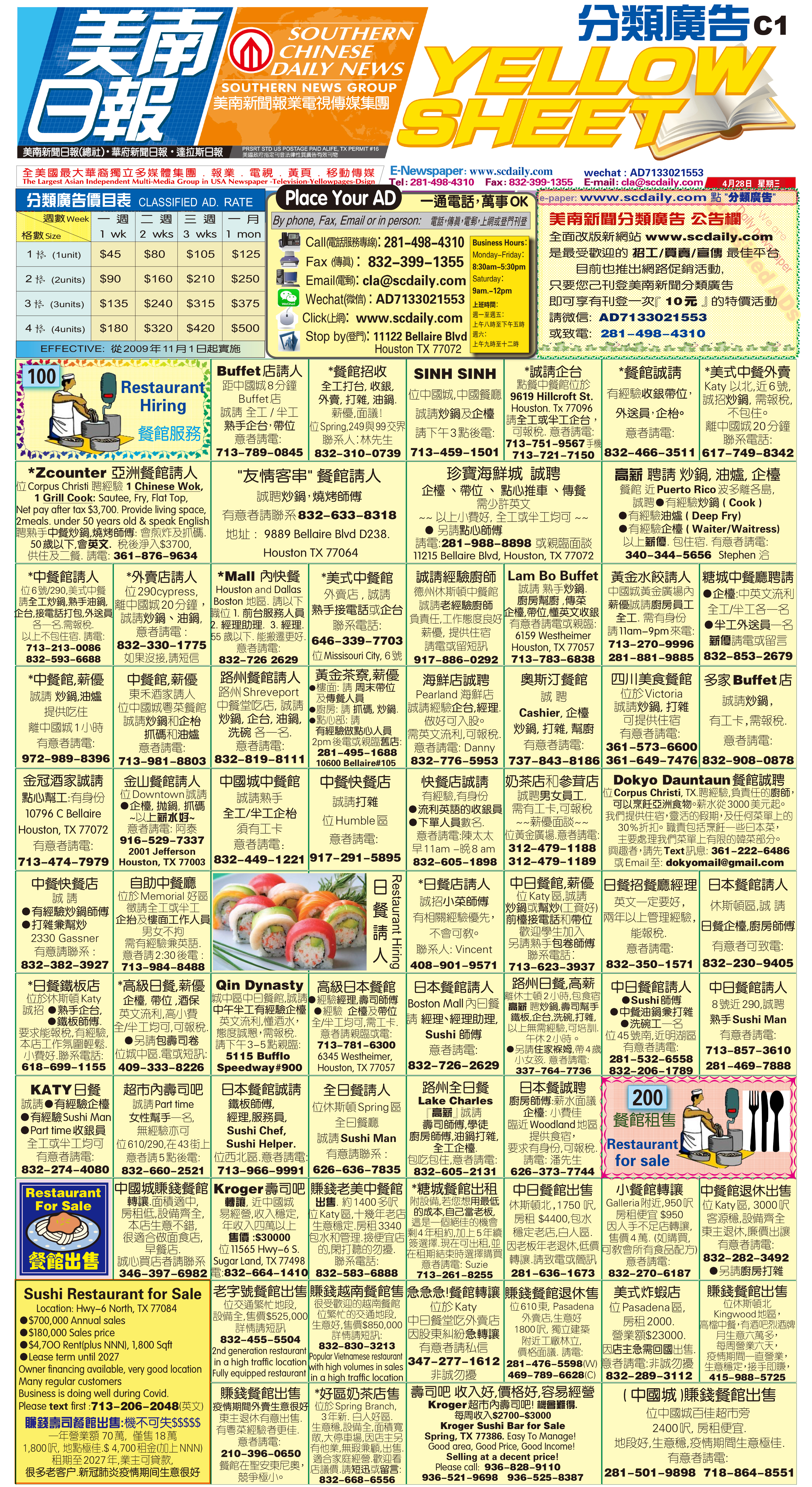210508 Epaper

 A Section B SectionC Section D Section210507 Epaper

 A Section B SectionC Section D Section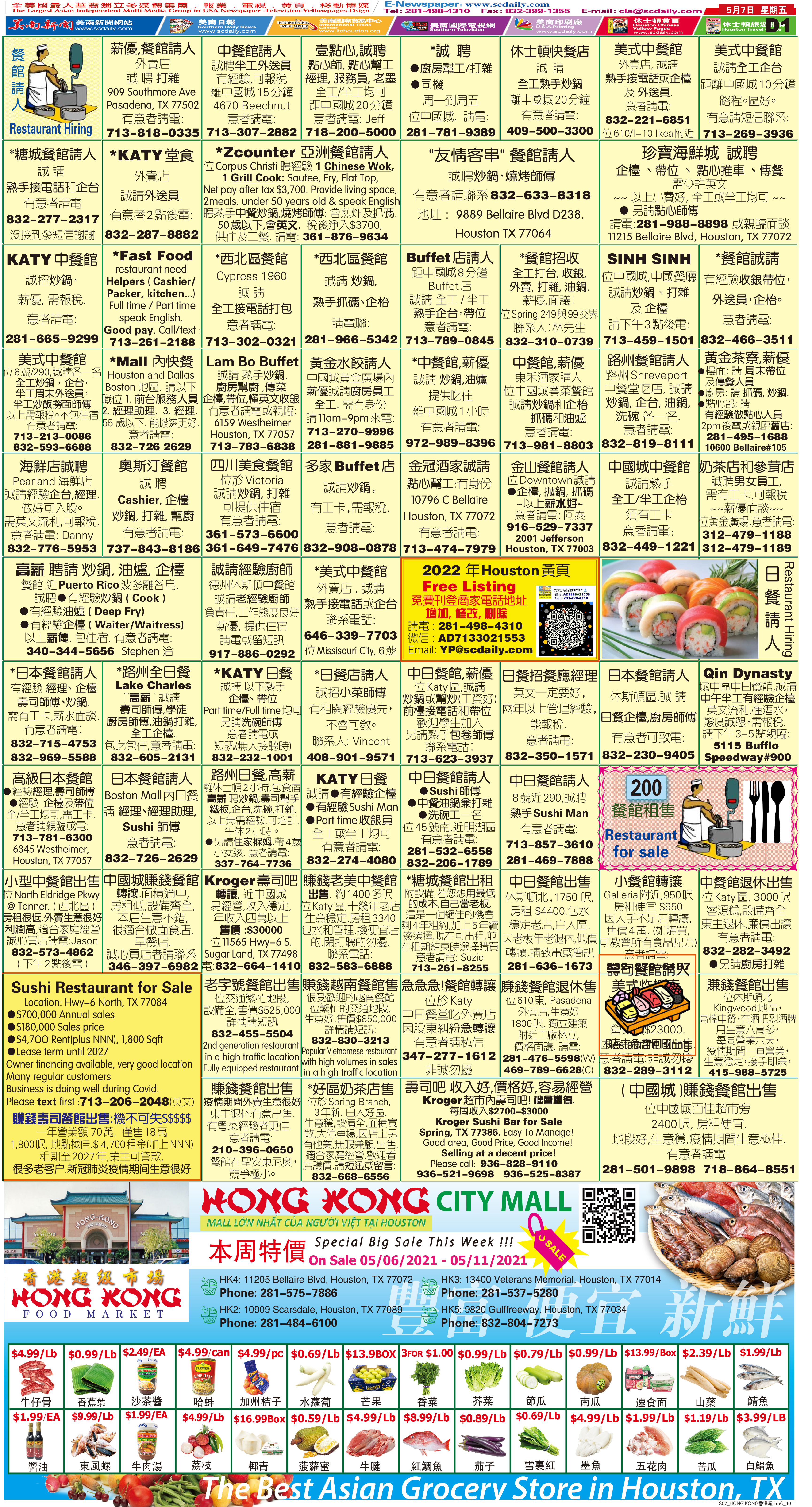210506 Epaper

 A Section B SectionC Section D Section210505 Epaper

 A Section B SectionC Section D Section210504 Epaper

 A Section B SectionC Section D Section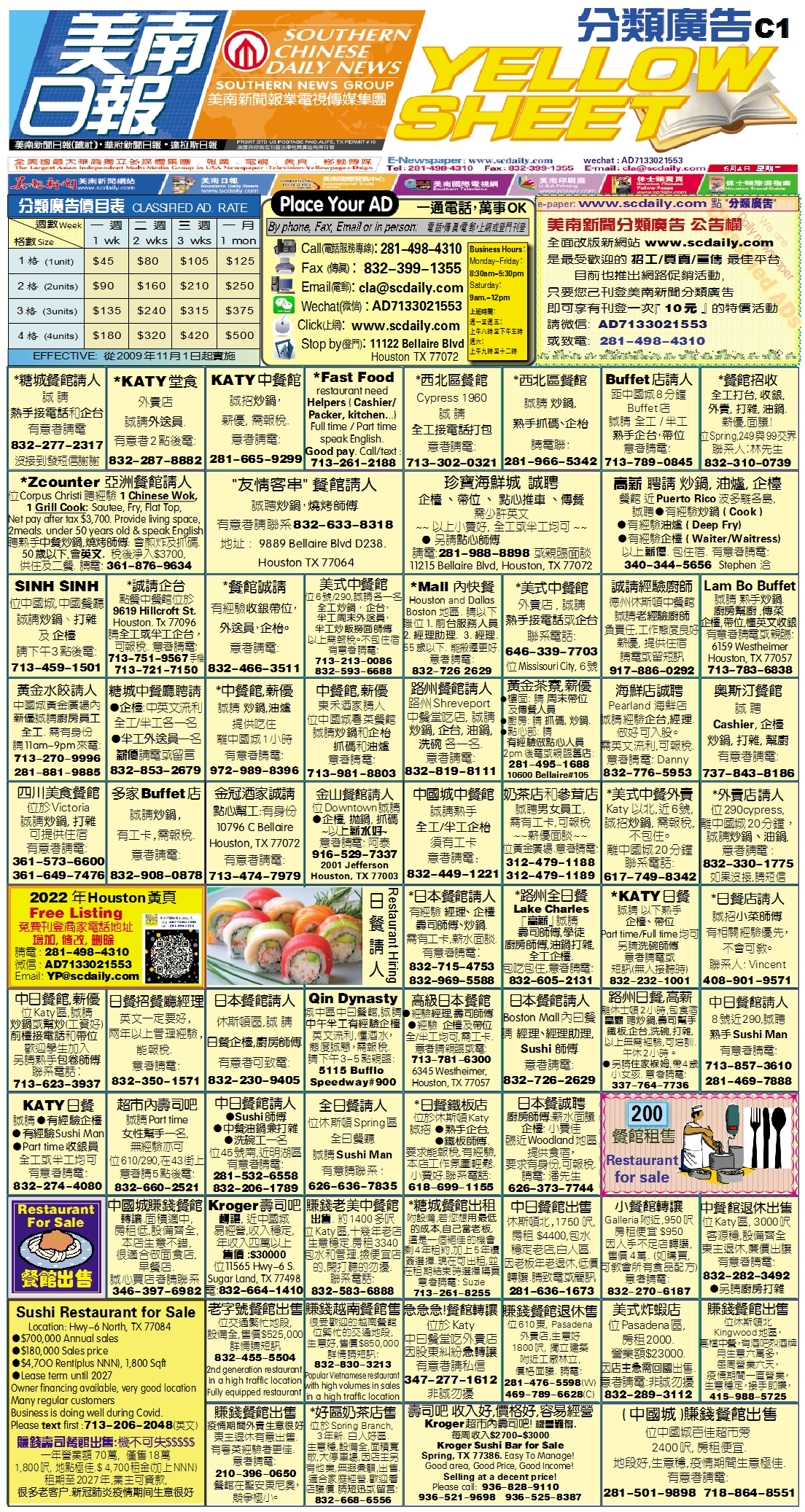210503 Epaper

 A Section B SectionC Section D Section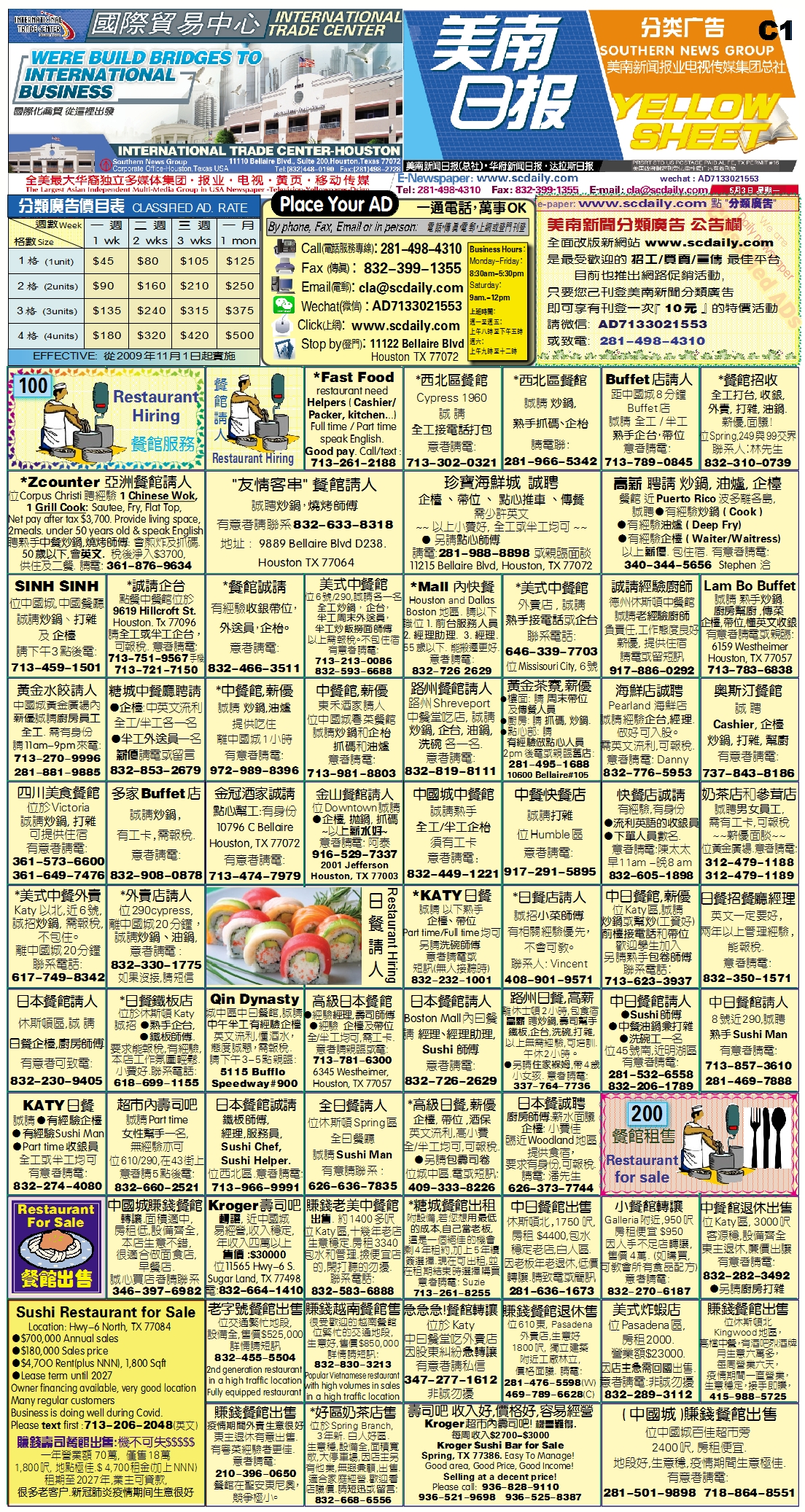210502 Epaper

 A Section B SectionC Section D Section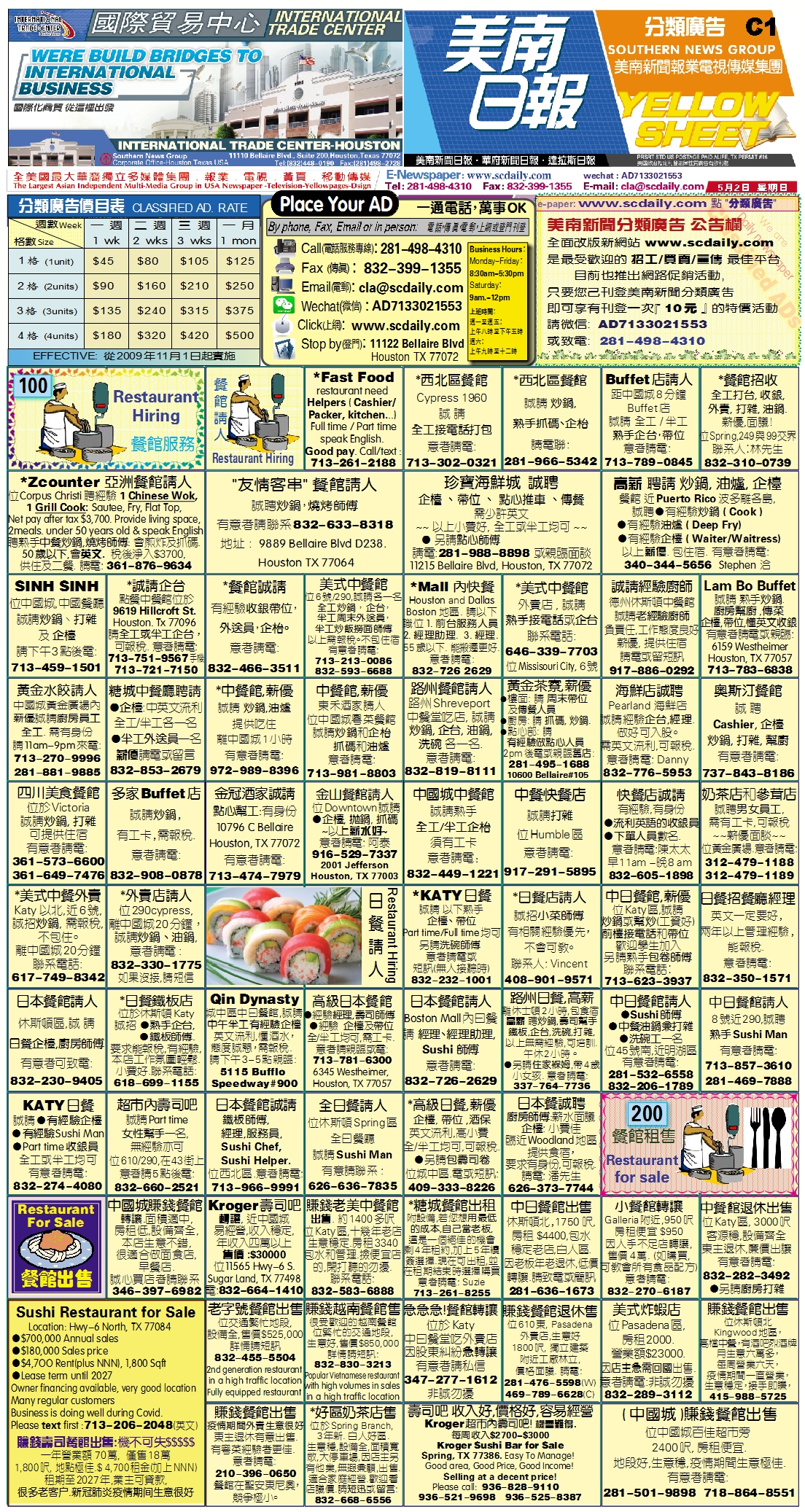210501 Epaper

 A Section B SectionC Section D Section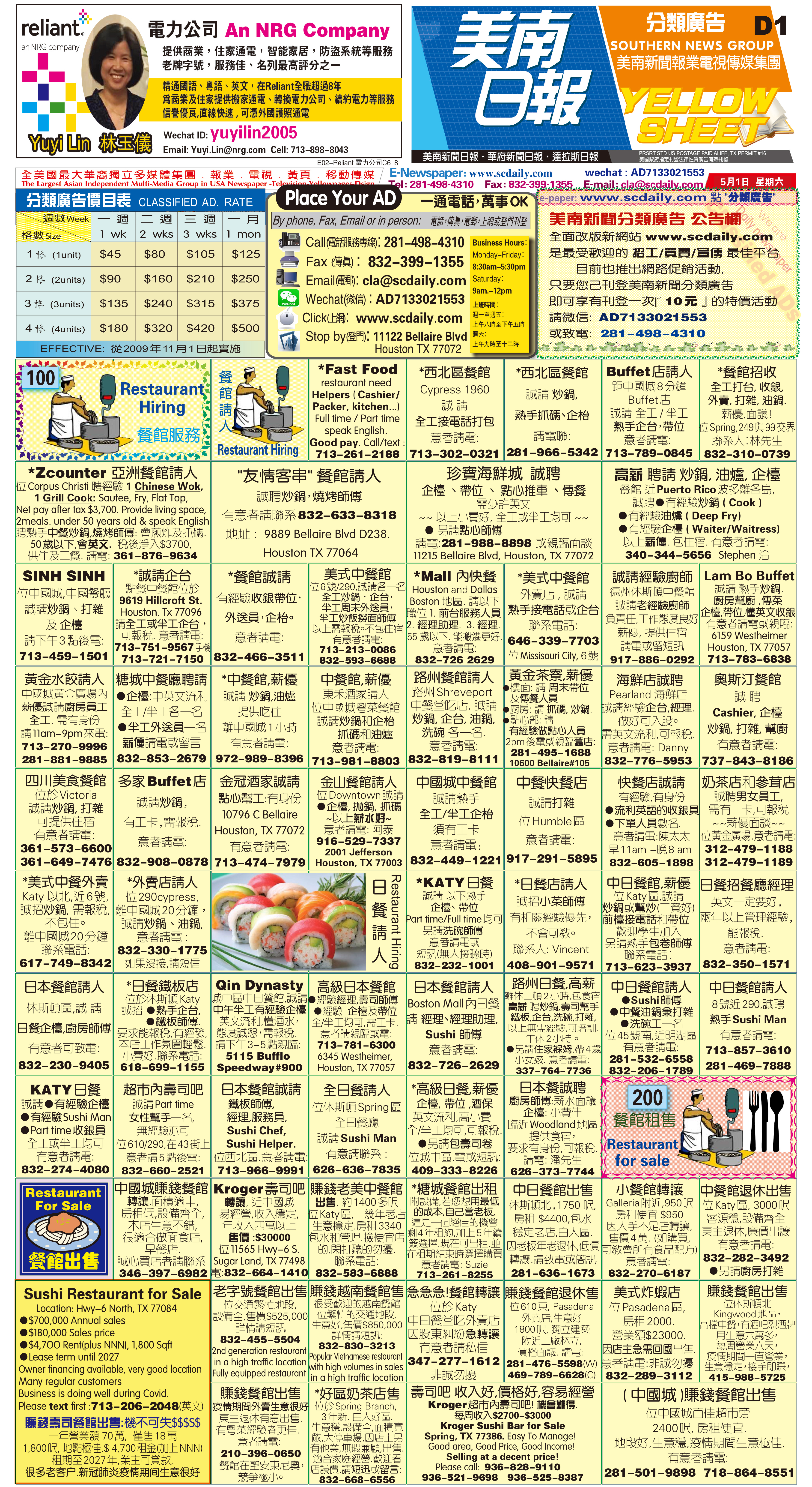210429 Epaper

 A Section B SectionC Section D Section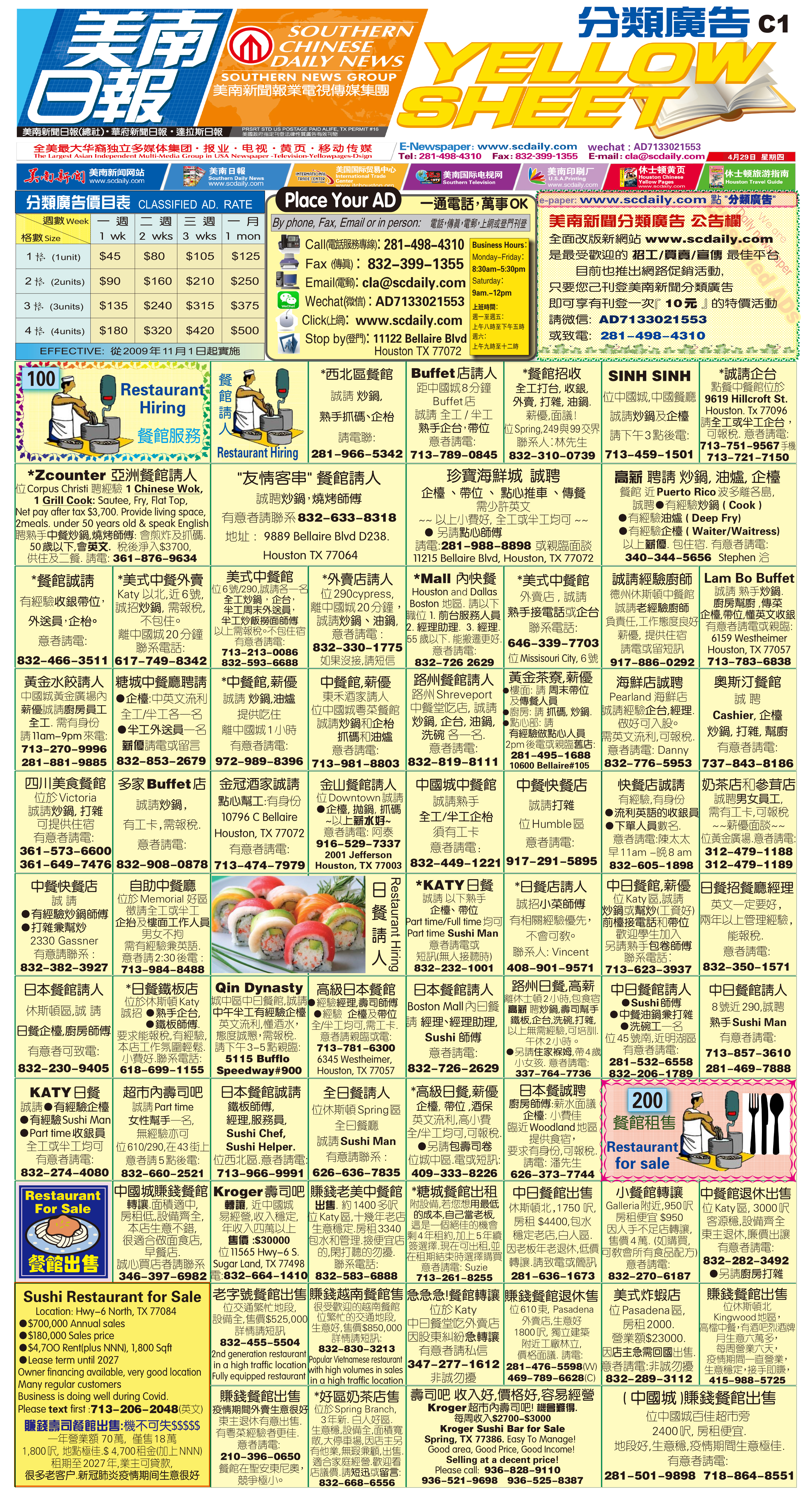210430 Epaper

 A Section B SectionC Section D Section210428 Epaper

 A Section B SectionC Section D Section